# PSEB 6th Class Maths Solutions Chapter 2 Whole Numbers Ex 2.1

Punjab State Board PSEB 6th Class Maths Book Solutions Chapter 2 Whole Numbers Ex 2.1 Textbook Exercise Questions and Answers.

## PSEB Solutions for Class 6 Maths Chapter 2 Whole Numbers Ex 2.1

Question (a)
Write the smallest whole number.
Solution:
The smallest Whole number = 0Question (b)
Write the smallest natural number.
Solution:
The smallest natural number = 1

Question (c)
Write the successor of 0 in whole numbers.
Solution:
Successor of 0 = 0 + 1 = 1

Question (d)
Write the predecessor of 0 in whole numbers.
Solution:
Predecessor of 0 is whole number is not possible.

Question (e)
Write the Largest whole number.
Solution:
Largest whole number is not possible.

2. Which of the following statements are True (T) and which are False (F)?

Question (a)
Zero is the smallest natural number.
Solution:
FalseQuestion (b)
Zero is the smallest whole number.
Solution:
True

Question (c)
Every whole number is a natural number.
Solution:
False

Question (d)
Every natural number is a whole number.
Solution:
True

Question (e)
1 is the smallest whole number.
Solution:
False

Question (f)
The natural number 1 has no predecessor in natural numbers.
Solution:
True

Question (g)
The whole number 1 has no predecessor in whole numbers.
Solution:
FalseQuestion (h)
Successor of the largest two-digit number is smallest three-digit number.
Solution:
True

Question (i)
The successor of a two-digit number is always a two-digit number.
Solution:
False

Question (j)
300 is the predecessor of 299.
Solution:
False

Question (k)
500 is the successor of 499.
Solution:
True

Question (l)
The predecessor of a two-digit number is never a single-digit number.
Solution:
False

3. Write the successor of each of following:

Question (a)
100909
Solution:
Successor of 100909
= 100909 + 1
= 100910Question (b)
4630999
Solution:
Successor of 4630999
= 4630999 + 1
= 4631000

Question (c)
830001
Solution:
Successor of 830001
= 830001 + 1
= 830002

Question (d)
99999.
Solution:
Successor of 99999
= 99999 + 1
= 100000

4. Write the predecessor of each of following:

Question (a)
1000
Solution:
Predecessor of 1000 = 1000 – 1
= 999Question (b)
208090
Solution:
Predecessor of 208090 = 208090 – 1
= 208089

Question (c)
7654321
Solution:
Predecessor of 7654321 = 7654321 – 1
= 7654320

Question (d)
12576.
Solution:
Predecessor of 12576 = 12576 – 1
= 12575

5. Represent the following numbers on the number line: 2, 0, 3, 5, 7, 11, 15.
Solution:
Draw a line. Mark a point on it. Label it ‘O’. Mark a second point to the right of 0. Label it 1. The distance between these points labelled as 0 and 1 is called unit distance. On this line, mark a point to the right of 1 and at unit distance from 1 and label it 2. In this way go on labeling points at unit distance as 3, 4, 5, …………… on the line.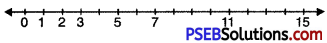6. How many whole numbers are there between 22 and 43?
Solution:
Whole numbers between 22 and 43 are 23, 24, 25, 26, 27, 28, 29, 30, 31, 32, 33, 34, 35, 36, 37, 38, 39, 40, 41, 42
∴ There are 20 whole numbers between 22 and 43.
Or [(43 – 22) – 1 = 21 – 1 = 20].7. Draw a number line to represent each of following on it.

Question (a)
3 + 2
Solution:
We draw a number line and move 3 steps from 0 to the right and mark this point as A.
Now, starting from A we move 2 steps towards right and move at B.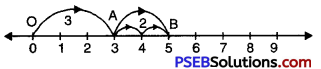OA = 4, AB = 2, OB = 5
Hence, OB = 3 + 2 = 5.

Question (b)
4 + 5
Solution:
We draw a number line.
Starting from point 0 (i.e. zero), we move 4 steps to the right and mark this point as A.
Now, starting from A we move 5 steps towards right and arrive at B.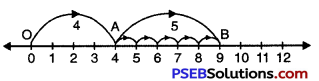OA = 4, AB = 5, OB = 9
Hence, OB = 4 + 5 = 9.Question (c)
6 + 2
Solution:
We draw a number line.
Starting from point 0 (i.e. zero), we move 6 steps to the right and mark this point as A.
Now, starting from A we move 2 steps towards right and arrive at B.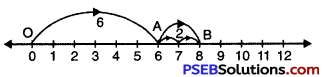OA = 6, AB = 2, OB = 8
Hence, OB = 6 + 2 = 8.

Question (d)
8 – 3
Solution:
We draw a number line.
Starting from point 0 (i.e. zero), we move 8 steps to the right and arrive at A.
Now, starting from A we move 3 steps to the left of A and arrive at B.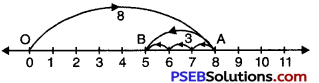OA = 8, AB = 3, OB = 5
Hence, OB = 8 – 3 = 5.

Question (e)
7 – 4
Solution:
We draw a number line.
Starting from point 0 (i.e. zero), we move 7 steps to the right and arrive at A.
Now, starting from A we move 4 steps to the left of A and arrive at B.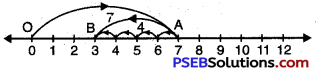OA = 7, AB = 4, OB = 3
Hence, OB = 7 – 4 = 3.Question (f)
7 – 2
Solution:
We draw a number line.
Starting from point 0 (i.e. zero), we move 7 steps to the right and arrive at A.
Now, starting from A, we move 2 steps to the left of A and arrive at B.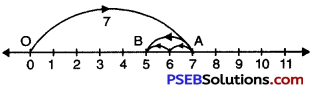OA = 7, AB = 2, OB = 5
Hence, OB = 7 – 2 = 5.

Question (g)
3 × 3
Solution:
We draw a number line.
Starting from 0 we move 3 units to the right of 0 to arrive at A.
We make two more such same moves starting from A (total 3 moves of 3 units each) to reach finally at C which represents 9.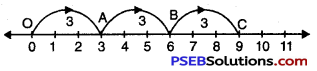Hence, 3 × 3 = 9.

Question (h)
2 × 5
Solution:
We draw a number line.
We start from 0 move 5 units at a time to right.
We make 2 such moves. We shall reach at 10.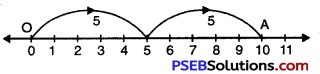So, 2 × 5 = 10.Question (i)
3 × 5
Solution:
We draw a number line.
We start from 0, move 5 units at a time to right.
We make 3 such moves. We shall reach at 15.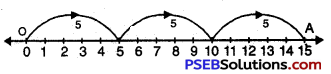So, 3 × 5 = 15

Question (j)
9 ÷ 3
We draw a number line.
Starting from 0, we move 9 units to the right of 0 to arrive at A.
Now, from A take moves of 3 units to the left of A till we reach at ‘O’. We observe that there are 3 moves.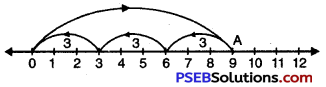So, 9 ÷ 3 = 3.

Question (k)
12 ÷ 4
We draw a number line.
Starting from 0, we move 12 units to the right of 0 to arrive at A.
Now, from A take moves of 4 units to the left of A till we reach at ‘O’. We observe that there are 3 moves.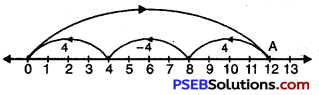So, 12 ÷ 4 = 3.Question (l)
10 ÷ 2
Solution:
We draw a number line.
Starting from 0, we move 10 units to the right of 0 to arrive at A.
Now, from A take moves of 2 units to the left c A till we reach at ‘O’. We observe that there are 5 moves.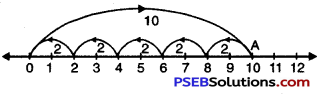So, 10 ÷ 2 = 5.

8. Fill in the blanks with the appropriate symbol < or > :

Question (i)
(a) 25 ……………. 205
(b) 170 …………… 107
(c) 415 …………… 514
(d) 10001 ………….. 9999
(e) 2300014 ………….. 2300041
(f) 99999 …………… 888888.
Solution:
(a) 25 < 205 (b) 170 > 107
(c) 415 < 514 (d) 10001 > 9999
(c) 2300014 < 2300041
(f) 99999 < 888888.